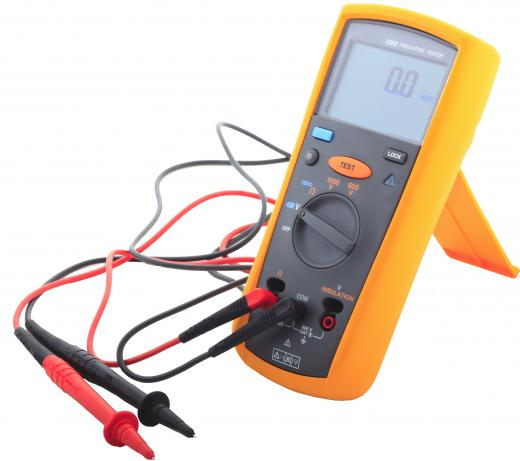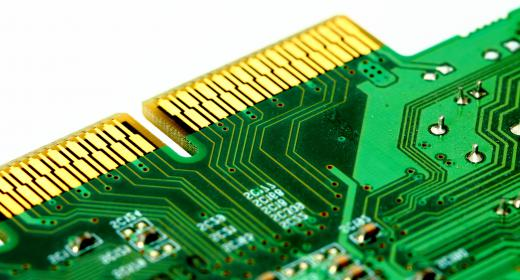Industry
Fact Checked

# What is Circuit Testing?

C.B. Fox
C.B. Fox

When electronic devices malfunction, there are ways to find the cause of the problem. Circuit testing is often the first diagnostics performed on an electrical device that has stopped working. A quick and relatively easy test to run, circuit testing can be performed with simple tools such as a multimeter or a test light. One can also use a more complex tool such as the In-Circuit Test.

Circuit testing determines whether electrical current can travel through a circuit. Resistance, measured in ohms, is the measure of the resistance in a circuit. A measure of 0 ohms means there is no resistance. Current flows freely with no obstacles. If the reading is infinite ohms means there may be a unintended break in the connection.A digital multimeter is a tool that can measure amps, volts, and ohms in a circuit.

The multimeter is a common tool used in circuit testing. Multimeters have two probes and either a digital or analog display. This simple device is used to determine whether electrical current is traveling from one end of a circuit to another. If the current reaches its destination, the multimeter will register that there are 0 ohms because there is no break in the circuit, or no resistance. If current cannot pass through, the device will register that there are infinite ohms which means that the electrical current is not traveling from one end to the other.Circuit testing is generally the first diagnostics performed on an electronic device that has stopped working.

Test lights can also be used to determine whether electricity is passing through a circuit. The principle in the test light is the same as that of the multimeter. Two electrodes, which are attached to a light bulb, are inserted into a circuit. These probes can be placed at any two points in the circuit that are supposed to conduct electricity. The bulb will light up if there is continuity between both connecting points. Many of these tools are made with sharp probes, allowing a circuit test to be performed through an insulated wire.

A more complex tool used in circuit testing is the In-Circuit Test (ICT). This tool determines whether electricity is passing through all the components on a circuit board properly, either to make sure the board was built correctly or to determine whether any damage was done to it. These tools contain a number of testers, sometimes up to 1000, that transmit information to a computer for analysis.

## You might also Like